21.1 (EAP/Beta)
20.2
20.1
19.2
19.1
18.2
18.1
17.2

# Cell Data Types

Each cell in a worksheet has a value that is specified by the CellValue object. To access this object, use the Range.Value property. A cell value is determined by the data contained within the cell:

A cell value can be of one of the following types: empty, numeric, text, Boolean or error. Cell values may have various display formats. For example, a numeric value can be displayed as a decimal number, a percentage or currency value, a date or time value, etc.

Use the properties of the CellValue object returned by the Range.Value property to retrieve information about the cell value type, and get the cell value itself as an object of the corresponding type. To get the string specifying the formatted value as it is displayed in a cell, use the Cell.DisplayText property.

The table below lists the available cell value types and provides examples on how a value of each type can be input, formatted, displayed and obtained.

Cell Value Type

Cell Content

Sample Input

Sample Display Format

Displayed String

Identify the Type

Obtain the Cell Value Object

Empty

The default cell value type.

If a cell contains any data, you can assign an empty value to it by setting the Range.Value property to null or CellValue.Empty.

For details, refer to the How to: Clear Cells of Content, Formatting, Hyperlinks and Comments document.

cell.Value = null

• or -

cell.Value = CellValue.Empty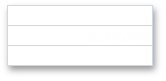CellValue.IsEmpty = true

CellValue.Empty

Numeric

The cell’s Range.Value property is assigned to a value of any numeric type (e.g., Int32, Double, etc.).

• or -

The cell’s Range.Formula property is assigned to an expression that returns a number.

cell.Value = 12345678

• or -

cell.Formula = “= SUM(12000000,345678)”

cell.NumberFormat = “#,#”

More examples:

How to: Specify Number or Date Format for Cell Content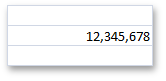CellValue.IsNumeric = true

CellValue.NumericValue

Numeric

(Date and Time)

The cell’s Range.Value property is assigned to the DateTime object, an object returned by the CellValue.FromDateTime method, or a numeric value representing a serial number of a date or time.

• or -

The cell’s Range.Formula property is assigned to an expression that returns the serial number of a date or time.

For details, refer to the Dates and Times in Cells document.

cell.Value = new DateTime(2012, 12, 10);

• or -

workbook.DocumentSettings.Calculation.Use1904DateSystem = true;

cell.Value = CellValue.FromDateTime(new DateTime(2012, 12, 10), true);

• or -

cell.Value = 41253;

• or -

cell.Formula = “=DATE(2012,12,10)”;

cell.NumberFormat = “m/d/yy”

More examples:

How to: Specify Number or Date Format for Cell Content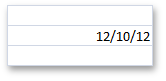CellValue.IsNumeric = true

CellValue.IsDateTime = true

CellValue.DateTimeValue

CellValue.NumericValue

Text

The cell’s Range.Value property is assigned to the String object.

• or -

The cell’s Range.Formula property is assigned to an expression that returns text.

cell.Value = “Sample Text”

• or -

cell.Formula = “= PROPER(“sample text”)”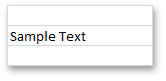CellValue.IsText = true

CellValue.TextValue

Boolean

The cell’s Range.Value property is assigned to the Boolean object.

• or -

The cell’s Range.Formula property is assigned to an expression that returns TRUE or FALSE.

cell.Value = true

• or -

cell.Formula = “= TRUE()”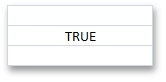CellValue.IsBoolean = true

CellValue.BooleanValue

Error

The cell’s Range.Value property is assigned to the CellValue object returned by the CellValue.Error* field (for example, CellValue.ErrorDivisionByZero, CellValue.ErrorInvalidValueInFunction, CellValue.ErrorName, etc.).

• or -

The cell’s Range.Formula property is assigned to an error code (e.g., “=#DIV/0!”, “=#N/A”, etc.) or an expression that cannot be calculated correctly (for example, an expression containing an invalid function name, value, division by zero, etc.)

For details, refer to the Error Types document.

cell.Value = CellValue.ErrorDivisionByZero

• or -

cell.Formula = “= #DIV/0!”

• or -

cell.Formula = “=5/0”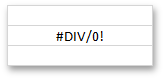CellValue.IsError = true

CellValue.ErrorValue